Courses

# Law Of Equivalents - Redox Reactions Chemistry Notes | EduRev

## Physical Chemistry

Created by: Asf Institute

## Chemistry : Law Of Equivalents - Redox Reactions Chemistry Notes | EduRev

The document Law Of Equivalents - Redox Reactions Chemistry Notes | EduRev is a part of the Chemistry Course Physical Chemistry.
All you need of Chemistry at this link: Chemistry

4. LAW OF EQUIVALENTS

Before heading for the law of equivalents, let us first discuss certain definitions.

4.1 MOLARITY (M)

It is defined as the number of moles of solute present in one litre of solution.

Molarity (M) =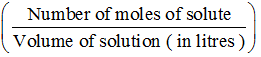Let the weight of solute be w g, molar mass of solute be Mg/mol and the volume of solution be V litre.

Number of moles of solute =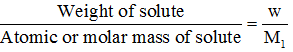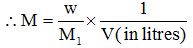∴ Number of moles of solute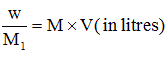4.2   NORMALITY (N)

It is defined as the number of equivalents of a solute present in one litre of solution equivalent is also the term used for amount of substance like mole with the difference that one equivalent of a substance in different reactions may be different as well as the one equivalent of each substance is also different.

Normality(N) =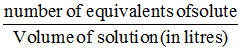Let the weight of solute be w g, equivalent mass of solute be E g/eqv. And the volume of solution be V litre.

Number of equivalents of solute=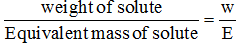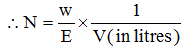Number of equivalents of solute=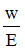= N x V (in liter)

4.3  EQUIVALENT MASS

Equivalent Mass =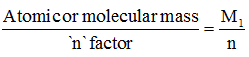∴ Number of equivalent solute =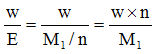∴ Number of equivalent os solute = n x number of moles of solute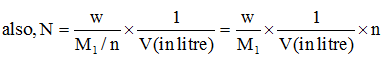N = M x n

∴ Normality of solution = n x molarity of solution

4.4 DILUTION EFFECT

When a solution is diluted, the moles and equivalents of solute do not change but and normality changes while on taking out a small volume of solution from a larger volume, the molarity and normality of solution do not change but moles and equivalents change proportionately.

In stoichiometry, the biggest problem is that for solving a problem we need to know a balanced chemical reaction. Since the number of chemical reactions are too many, it is not possible to remember all those chemical reactions. So, there is need to develop an approach which does not require the use of balanced chemical reaction. This approach makes use of a law called law of equivalence. The law of equivalence provides us the molar ratio of reactants and products without knowing the complete balanced reaction, which is as good as having a balanced n-factor of relevant species.

According to the law of equivalence, whenever two substances react, the equivalents of one will be equal to the equivalents of other and the equivalents of any product will also be equal to that of the reactant.

Let us suppose we have a reaction, A + B→C + D. In this reaction, the number of moles of electrons lost by 1 mole of A are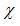and the number of electrons gained by 1 mole of B are y. since, the number of mole of electrons lost and gained are not same, the molar ratio in which A and B react cannot be 1:1. Thus, if we take y moles of A, then the total moles of electrons lost by y moles of A would be. Similarly, if x moles of B are taken, then the total mole of electrons gained by x moles of B would beThus the number of electrons lost by A and number of electrons gained by B becomes equal. For reactant A, its n-factor is x and the number of moles used are y.  So,

The equivalent of A reacting = moles of A reacting x n-factor of A=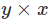Similarly, for react ant B, its n-factor is y and the number of moles used are x. so,

The equivalents of B reacting= moles of B reacting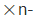factor of B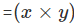Thus the, equivalents of A reacting would be equal to the equivalents of B reacting. Thus, the balancing coefficients of the reactant would be as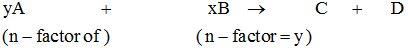The n-factor of A and B are in the ratio of x:y, and their molar ratio is y:x. thus, molar ratio is inverse of the n-factor ratio.

In general, whenever two substances react with their n-factors in the ratio of a:b, then their molar ratio in a balanced chemical reaction would be b:a.

To get the equivalents of a substance, its n-factor has to be known. Let the weight of the substance used in the reaction be w g.

Then, equivalents of substance reacted would be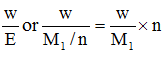(where E and M1 are the equivalent mass and molar mass of the substance) thus, in order to calculate the equivalents of substance, knowledge of n-factor is a must (which we will be dealing in section-II ).

Offer running on EduRev: Apply code STAYHOME200 to get INR 200 off on our premium plan EduRev Infinity!

## Physical Chemistry

77 videos|83 docs|32 tests

,

,

,

,

,

,

,

,

,

,

,

,

,

,

,

,

,

,

,

,

,

;